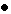III. CHARACTERIZATION Given a set of data of the fractional frequency or time fluctuations between a pair of oscillators, it is useful to characterize these fluctuations with reasonable and tractable models of performance. In so doing for many kinds of oscillators, it is useful to consider the fluctuations as those that are random (may only be predicted statistically) and those that are non-random (eg., systematics--those that are environmentally induced or those that have a causal effect that can be determined and in many cases can be predicted). 3.1 Non-random Fluctuations Non-random fluctuations are usually the main cause of departure from "true" time or "true" frequency in the long term. If, for example, one has the values of the frequency over a period of time and a frequency offset from nominal is observed, one may calculate directly that the phase error will accumulate as a ramp. If the frequency values show some linear drift then the time fluctuations will depart as a quadratic. In almost all oscillators, the above systematics, as they are sometimes called, are the primary cause of time and/or frequency departure. A useful approach to determine the value of the frequency offset is to calculate the simple mean of the set, or for determining the value of the frequency drift by calculating a linear least squares fit to the frequency. A least squares quadratic fit to the phase or to the time deviations is typically not as efficient an estimator of the frequency drift for most oscillators. Precision frequency standards are affected by their environment. These environmental purturbations often cause long-term frequency and time departures. 3.2 Random Fluctuations After calculating or estimating the systematic or non-random effects of a data set, these may be subtracted from the data leaving the residual random fluctuations. These can usually be best characterized statistically. It is often the case for precision oscillators that these random fluctuations may be well modeled with power law spectral densities. This topic is discussed later in sections VIII to X. We have Sy(f) = ha fa (3.1) where Sy(f) is the one-sided spectral density of the fractional frequency fluctuations, f is the Fourier frequency at which the density is taken, ha is the coefficient indicating the level of that type of noise, and a is a number modeling the most appropriate type of power law for the data. The agreed upon standard (IEEE) in the time domain is s y(t ), to be explained in the next section. If one observes from a log s y2(t ) versus log t diagram a particular slope (call it m ) over certain regions of sample time, t , this slope has a correspondence to a power law spectral density or a set of the same with some amplitude coefficient ha . In particular, m = -a -1 for -3 < a < 1 and m =- a -2 for 1 > a . Further a correspondence exists between ha and the coefficient for s y(t ). These coefficients have been calculated and appear in section XI. The transformations for some of the more common power law spectral densities have been tabulated making it quite easy to transform the frequency stability modeled in the time-domain over to the frequency domain and vice versa. Examples of some power-law spectra that have been simulated by computer are shown in figure 3.1. In descending order these have been named white noise, flicker noise, random walk, and flicker walk (the w in fig. 3.1 is angular Fourier frequency, w = 2p f). Once the noise characteristics have been determined, one is often able to deduce whether the oscillators are performing properly or not and whether they are meeting either the design specifications or the manufacturers specifications. For example a cesium beam frequency standard or a rubidium gas cell frequency standard when working properly should exhibit white frequency noise which is the same as random walk phase (or time) for tau values of the order of a few seconds to several thousand seconds (see also sec. XI).Main PageTable of ContentsGo to section: Summary and IntroductionIIIIIIIVVVIVIIVIIIIXXXIXIIConclusionReferences